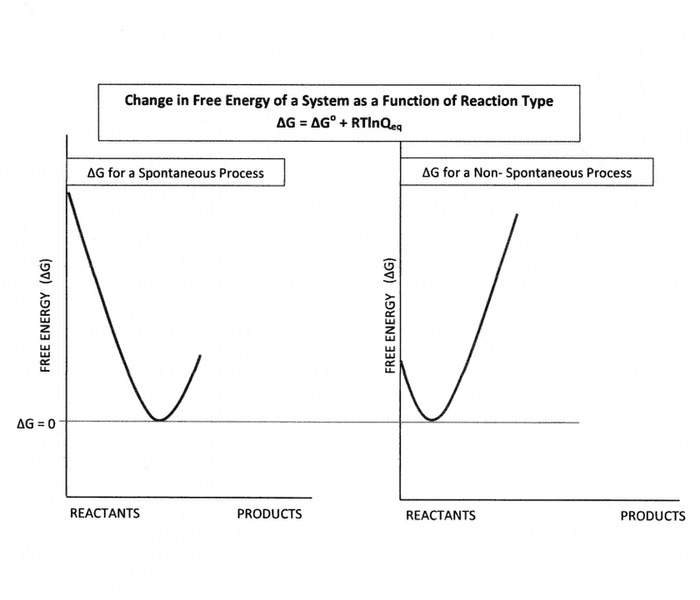# Is the minimum Gibbs free energy the same for every material?

• ItDoesn'tMatter
In summary, the minimum absolute free energy for a material is the same for every material. This is based on the equation ΔG = ΔGo + RTlnQeq, where ΔG defines a system under non-standard conditions and is always tending towards the most stable condition of disorder. However, different substances can have different changes in standard free energies, implying that the more negative the standard free energy, the more stable the system. Outside of standard state conditions, all systems tend to ΔG = 0. This can be a controversial topic and any rebuttals are welcome.

#### ItDoesn'tMatter

We were learning about the change in free energy in class, but I was wondering since the Gibbs free energy decreases as a system becomes more stable, is the minimum absolute free energy for a material the same for every material, or can other materials be more stable than others?

ItDoesn'tMatter said:
is the minimum absolute free energy for a material the same for every material,
Hmmmm, interesting question... I'd say 'yes' if your defense is based upon ΔG = ΔGo + RTlnQeq. The ΔG in this equation defines a system under 'non-standard' conditions. When ΔG ≠ 0 the system is dynamic in nature and its free energy can change based upon whether or not the system is spontaneous or non-spontaneous in nature, but still indicates the system of interest is tending always to the most stable condition of disorder. See attached figure. This most 'stable' condition occurs when ΔG = 0 the the system is said to be at its minimum free energy and is defined by ΔG = 0 = ΔGo + RTlnQeq. This is the minimum ΔG that can occur for any system. However, the equation also shows that at ΔG = 0 => ΔGo = -RTlnKeq = ∑n⋅ΔGfo(Products) - ∑n⋅ΔGfo(Reactants). This implies that different substances (reacting systems) have different changes in 'standard' free energies based upon the chemical and physical properties of the system of interest. Of course the more negative 'standard' free energy would be the more stable system but outside the standard state conditions, all systems tend to ΔG = 0 ...This is a double edged sword depending on how you define the free energy change of a system. I welcome any and all rebuttals to this point of view. It is an interesting issue. Thanks all. Doc
http://blob:https%3A//www.physicsforums.com/578ec8ce-71b9-44ed-bbfa-8a1bdf1f227b## What is Gibbs free energy?

Gibbs free energy is a thermodynamic quantity that represents the amount of energy available to do work in a system at constant temperature and pressure. It is denoted by the symbol G and is a combination of the system's internal energy and the energy that is released or absorbed during a process.

## How is Gibbs free energy related to material properties?

Gibbs free energy is a useful concept in materials science because it can be used to predict the direction of chemical reactions and phase changes in a material. It is also a factor in determining the stability of a material and its tendency to undergo transformations or reactions.

## What is the minimum Gibbs free energy?

The minimum Gibbs free energy is the lowest possible value that this quantity can take for a given material at a specific temperature and pressure. It represents the most stable state that the material can achieve under those conditions.

## Is the minimum Gibbs free energy the same for all materials?

No, the minimum Gibbs free energy is not the same for all materials. It is dependent on factors such as temperature, pressure, and the composition and structure of the material. Different materials will have different minimum Gibbs free energy values at the same conditions.

## How can the minimum Gibbs free energy be calculated?

The minimum Gibbs free energy can be calculated using thermodynamic equations and data, such as the enthalpy and entropy of a material. These calculations can be complex and require knowledge of the material's properties, but they can provide valuable insights into the behavior of a material under different conditions.